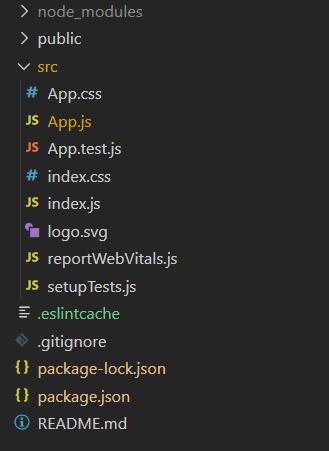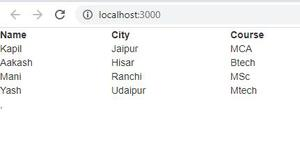# How to build an HTML table using ReactJS from arrays ?

• Last Updated : 07 Apr, 2021

If we have an array and want to build an HTML table of it using ReactJS we can use the map function. The map() method iterates through each element of the array and will convert it into a table row. First, we will create a table tag then first, we will iterate through the heading/column names of the table and convert them into a table header using the <th> tag. Then we will iterate through the table data and convert them into each row as a table body using the <td> tag.

Creating React Application:

• Step 1: Create a React application using the following command:

`npx create-react-app foldername`
• Step 2: After creating your project folder i.e. foldername, move to it using the following command:

`cd foldername`

Project Structure: It will look like the following.Example: Now write down the following code in the App.js file. Here, App is our default component where we have written our code.

## App.js

 `import React, { Component } from ``'react'``;`` ` `class App extends Component {``    ``render() {``        ``var` `heading = [``'Name'``, ``'City'``, ``'Course'``];``        ``var` `body =``            ``[[``'Kapil'``, ``'Jaipur'``, ``'MCA'``],``            ``[``'Aakash'``, ``'Hisar'``, ``'Btech'``],``            ``[``'Mani'``, ``'Ranchi'``, ``'MSc'``],``            ``[``'Yash'``, ``'Udaipur'``, ``'Mtech'``]``            ``];``        ``return` `(``            ``
``                ``,``            ``
``        ``);``    ``}``}`` ` `class Table extends Component {``    ``render() {``        ``var` `heading = ``this``.props.heading;``        ``var` `body = ``this``.props.body;``        ``return` `(``            ````                ``
``                    ````                        ``{heading.map(head => )}``                    ````                ````                ````                    ``{body.map(row => )}``                ````            ````        ``);``    ``}``}`` ` `class TableRow extends Component {``    ``render() {``        ``var` `row = ``this``.props.row;``        ``return` `(``            ``
{val}
``                ``{row.map(val => )}``            ````        ``)``    ``}``}`` ` `export ``default` `App;`

Step to Run Application: Run the application using the following command from the root directory of the project:

`npm start`

Output: Now open your browser and go to http://localhost:3000/, you will see the following output:As we can see from the output we use <th> tag for the heading and <td> tag for the remaining rows. The map function iterating through each row and returning a row, and it is adding to the table.

My Personal Notes arrow_drop_up